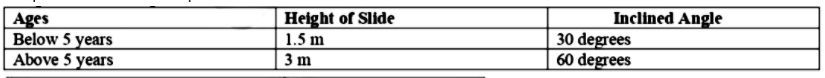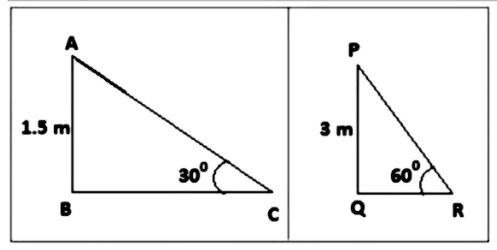Home/Class 10/Maths/

A contractor plans to install two slides for the children to play in a park. For the children below the age of $$5$$ years, she prefers to have a slide whose top is at a height of $$1.5\ m$$, and is inclined at an angle of $$30^o$$ to the ground, whereas for elder children, she wants to have a steep slide at a height of $$3\ m$$, and inclined at an angle of $$60^o$$ to the ground. What should be the length of the slide in each case?Speed
00:00
06:18## QuestionMathsClass 10

A contractor plans to install two slides for the children to play in a park. For the children below the age of $$5$$ years, she prefers to have a slide whose top is at a height of $$1.5\ m$$, and is inclined at an angle of $$30^o$$ to the ground, whereas for elder children, she wants to have a steep slide at a height of $$3\ m$$, and inclined at an angle of $$60^o$$ to the ground. What should be the length of the slide in each case?

As per contractor’s plan,Let, $$ABC$$ is the slide inclined at $$30^o$$ with length $$AC$$and $$PQR$$is the slide inclined at $$60^o$$ with length $$PR$$.
To Find: $$AC$$and $$PR$$
In right $$ΔABC$$,
$$\sin 30^o = \frac{AB}{AC}$$
$$\implies \frac{1}{2} = \frac{1.5}{AC}$$
$$\implies AC = 3$$
Also,in right $$ΔPQR$$,
$$sin 60^o = \frac{PQ}{PR}$$
$$\implies \frac{\sqrt{3}}{2}$$ $$= \frac{3}{PR}$$
$$\implies PR = 2$$$$\sqrt{3}$$
Hence, length of the slide for below $$5 = 3 \ m$$ and
Length of the slide for elders children $$= 2$$$$\sqrt{3} \ m$$